Courses

# Least Squares Fitting - Data Analysis, CSIR-NET Physical Sciences Physics Notes | EduRev

## Physics for IIT JAM, UGC - NET, CSIR NET

Created by: Akhilesh Thakur

## Physics : Least Squares Fitting - Data Analysis, CSIR-NET Physical Sciences Physics Notes | EduRev

The document Least Squares Fitting - Data Analysis, CSIR-NET Physical Sciences Physics Notes | EduRev is a part of the Physics Course Physics for IIT JAM, UGC - NET, CSIR NET.
All you need of Physics at this link: Physics

I. INTRODUCTION

Frequently we are given a set of data points (xi, yi ), i = 1, 2, . . . , N , through which we would like to t to a smooth function. The function could be straight line (the simplest case), a higher order polynomial, or a more complicated function. As an example, the data in Fig. 1 seems to follow a linear behavior and we may like to determine the "best" straight line through it. More generally, our tting function, y = f (x), will have some adjustable parameters and we would like to determine the "best" choice of those parameters.

The de nition of "best" is not unique. However, the most useful choice, and the one nearly always taken, is the "least squares" t, in which one minimizes the sum of the squares of the difference between the observed y-value, yi , and the tting function evaluated at xi , i.e.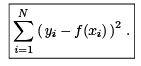(1)

The simplest cases, and the only ones to be discussed in detail here, are where the tting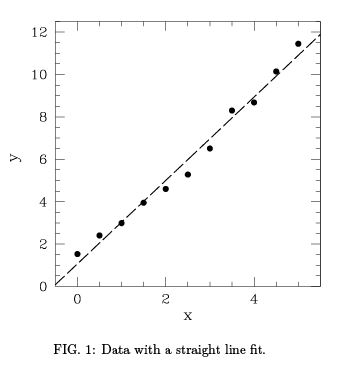function is a linear function of the parameters. We shall call this a linear model. Examples are a straight line

y = a0 + a1x                                   (2)

and an m-th order polynomial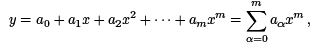(3)
where the parameters to be adjusted are the aα. (Note that we are not requiring that y is a linear function of x, only of the t parameters aα .)

An example where the tting function depends non -linearly on the parameters is

y = a0xa1 + a2,                            (4)

Linear models are fairly simply because, as we shall see, the parameters are determined by linear equations, which always have a unique solution which can be found by straightforward methods. However, for tting functions which are non-linear functions of the parameters, the resulting equations are non-linear which may have many solutions or non at all, and so are much less straightforward to solve.

Sometimes a non-linear model can be transformed into a linear model by a change of variables.
For example if we want to t to

y = a0xa1 ,             (5)

which has a non-linear dependence on a1, then taking logs gives

ln y = ln a0 + a1 ln x ,                      (6)

which is a linear function of the parameters a'0 = ln a0 and a1 . Fitting a straight line to a log-log plot is a very common procedure in science and engineering.

II. FITTING TO A STRAIGHT LINE

To see how least squares tting works, consider the simplest case of a straight line t, Eq. (2), for which we have to minimize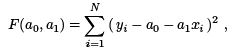(7)

with respect to aand a1. Differentiating F with respect to these parameters and setting the results to zero gives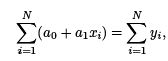(8a)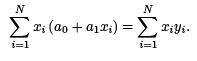(8b)

We write this as

U00 a0 + U01 a1 = v0,             (9a)

U10 a0 + U11 a1 = v1,               (9b)

where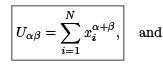(10)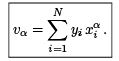(11)

Equations (9) are two linear equations in two unknowns. These can be solved by eliminating one variable, which immediately gives an equation for the second one. The solution can also be determined from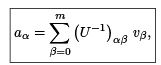(12)

where m = 1 here, and the inverse of the 2 x 2 matrix U is given, according to standard rules, by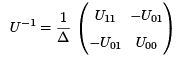(13)
where

(14)
and we have noted that U is symmetric so U01 = U10. The solution for a0 and a1 is therefore given by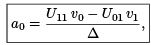(15a)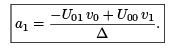(15b)

We see that it is straightforward to determine the slope, a1 , and the intercept, a0 , of the t from Eqs. (10), (11), (14) and (15) using the N data points (xi , yi ).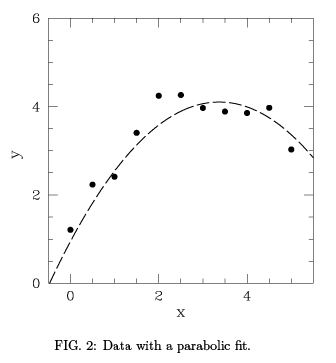III. FITTING TO A POLYNOMIAL

Frequently it may be better to t to a higher order polynomial than a straight line, as for example in Fig. 2 where the t is a parabola. Using the notation for an m-th order polynomial in Eq. (3), we need to minimize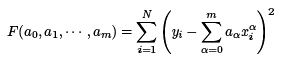(16)

with respect to the M = m + 1 parameters aα. Setting to zero the derivative of F with respect to aα gives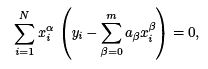(17)

which we write as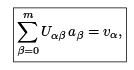(18)

where Uαβ   and vα  are de ned in Eqs. (10) and (11). Eq. (18) represents M = m + 1 linear equations, one for each value of α . Their solution is given formally by Eq. (12).

Hence polynomial least squares fits, being linear in the parameters, are also quite straightforward. We just have to solve a set of linear equations, Eq. (18), to determine the fit parameters.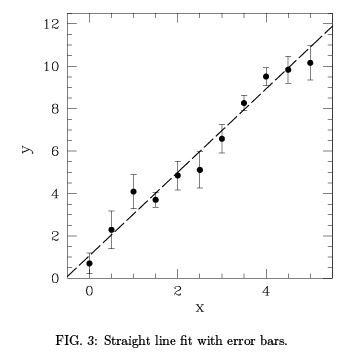IV. FITTING TO DATA WITH ERROR BARS

Frequently we have an estimate of the uncertainty in the data points, the "error bar". A fi t would be considered satisfactory if it goes through the points within the error bars. An example of data with error bars and a straight line t is shown in the fi gure below.

If some points have smaller error bars than other we would like to force the fit to be closer to those points. A suitable quantity to minimize, therefore, is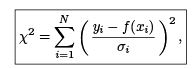(19)

called the "chi-squared" function, in which σi is the error bar for point i. Assuming a polynomial fit, we proceed exactly as before, and again find that the best parameters are given by the solution of Eq. (18), i.e.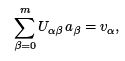(20)

but where now Uαβ   and vα are given by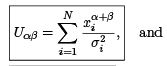(21)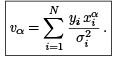(22)

The solution of Eqs. (20) can be obtained from the inverse of the matrix U , as in Eq. (12).
Interestingly the matrix U -1 contains additional information. We would like to know the range of values of the aα which provide a suitable fi t. It turns out, that the square of the uncertainty in aα is just the appropriate diagonal element of U -1 , so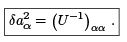(23)

For the case of a straight line fit, the inverse of U is given explicitly in Eq. (13). Using this information, and the values of (xi , yi , σi) for the data in the above gure, I find that the fit parameters (assuming a straight line fit) are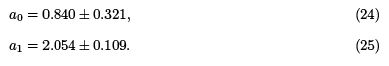I had generated the data by starting with y = 1 + 2x and adding some noise with zero mean. Hence the t should be consistent with y = 1 + 2x within the error bars, and it is.

V. CHI-SQUARED DISTRIBUTION

It is all very well to get the t parameters and their error bars, but these results don't mean much unless the fi t actually describes the data. Roughly speaking, this means that it goes through the data within the error bars. To see if this is the case, we look at the value X2 de ned by Eq. (19) with the optimal parameters. Intuitively, we expect that if the fi t goes through the data within about one error bar, then X2 should be about N . This indeed is correct, and, in fact, one can get much more information, including the distribution of X2 , if we assume that the data points yi have a Gaussian distribution.

Let us rst assume that, apart from the (Gaussian) noise, the data exactly ts a polynomial,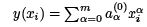for some parameters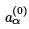. We rst calculate the distribution of X2 where we put in the exact values for the parameters,, rather than those obtained my minimizing X2 with respect to the parameters aα. (Afterwords we will consider the effect of minimizing with respect to the aα .) We therefore consider the distribution of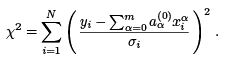(26)

Each of the N terms is the (square of) a Gaussian random variable with mean 0 and standard deviation unity. Denoting these variables by ti then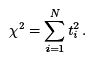(27)

Firstly, suppose that N = 1. The single variable t has the distribution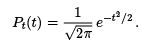(28)

We actually want the distribution of t2 . Let us call u = t2 . To get the distribution of u, which we call Pu (u), from the distribution of t, where u is a function of t, we note that the probability that t lies between t and t + dt is the probability that u lies between u and u + du where du=dt is the derivative, i.e.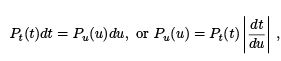(29)

where we noted that if the derivative is negative we must take the absolute value (since we are just equating the weight of the distribution of t over an interval dt to the weight of the distribution of u over an interval du). In this case we get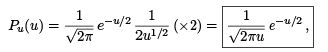(30)

where we also multiplied by 2 since there are two solutions for t of t2 = u. Remember Eq. (30) just corresponds to the Gaussian distribution in Eq. (28) but with u = t2 . It is instructive to check that the distribution in Eq. (30) is normalized.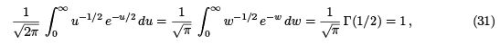where we made the substitution w = u/2 and used the result, discussed in class, that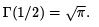.

As we have discussed, to obtain the distribution of a sum of random variables, such as in Eq. (27), it is useful to Fourier transform the distribution of the individual variables. We therefore take the Fourier transform of Pu(u),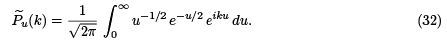With the substitution w = (1- 2ik)(u=2) we get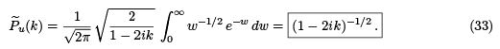Now X2 in Eq. (27) is just the sum of N random variables each of which has the distribution in Eq. (30). Hence the Fourier transform of the distribution of X2 is the N -th power of the Fourier transform of the distribution of one variable, which is given by Eq. (33), so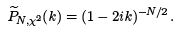(34)

We will now verify that the function whose Fourier transform gives this expression is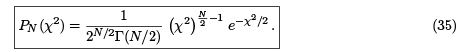(Note that we are determining the distribution of X2 , not X, we are following convention in writing the basic variable as the square of something.) The Fourier transform of Eq. (35) is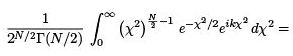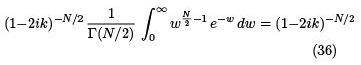which gives Eq. (34) as desired. We have again made the substitution w = (1 2ik)(X2=2), and we also used the de nition of the τ (N/2).
Hence the X2 distribution for N variables is given by Eq. (35). From this it is easy to determine the mean and standard deviation of X2, if we remember the de nition of the Gamma function: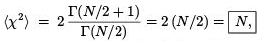(37)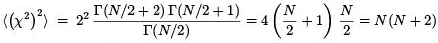(38)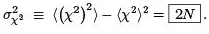(39)

Hence the average value of X2 is N (as we guessed intuitively above) and the standard deviation is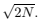In my opinion, it is often more convenient to consider the distribution of X2 =N which is called the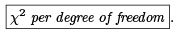This has mean unity (independent of N ) and standard deviation   isFigure 4 shows the distribution of X2 /N , the chi-squared per degree of freedom, for N = 2, 5 and 50. Note that for N = 2 the distribution of X2 / N , is an exponential, for N > 2 it vanishes at the origin, and for N → ∞ the central limit theorem tells us (as we can verify) that it is a Gaussian of mean 1 and standard deviation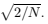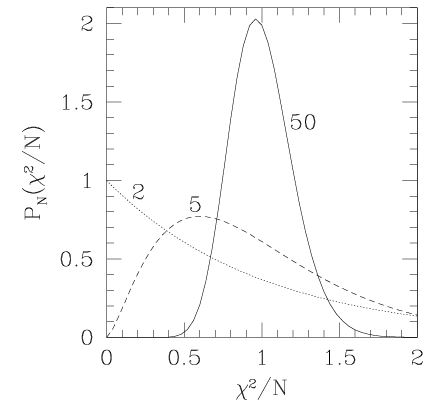FIG. 4: The chi-squared distribution per degree of freedom for N = 2, 5 and 50.

We have obtained the distribution of X2 in Eq. (26) assuming that data ts exactly the speci ed polynomial apart from the Gaussian noise. However, in practice, we do not know the polynomial and we estimate it by minimizing X2 in Eq. (19) with respect to the parameters aα. It turns out that the net effect is to decrease the "number of degrees of freedom", which was N before, by the number of parameters in the t M (so for example M = m + 1 for an m-th order polynomial t).
To show this precisely requires some rather heavy math, which I will avoid, but instead indicate intuitively how the result arises.

Consider the simple case of a straight line t, y = a0 + a1x. Suppose we added a constant to all the yi. Clearly X2 would be unchanged once I've minimize with respect to abecause the modi ed value of a0 would exactly compensate for the shift in the y values. Similarly, if I change the yi by an amount which is proportional to the xi then again the X2 would be unchanged because the new value for a1 would exactly compensate for the change in the data. Hence only changes in the yi which are orthogonal to δyi = c0, and δyi = c1xi give a change in X2 after minimization. There are N - 2 such linear combinations. It turns out that each gives a contribution to X2 with the same distribution as each of the terms in the unoptimized case, i.e. Eq. (30).

Hence, when we minimize X2 to get the best t, if the t were perfect apart from the random Gaussian noise in the data, the distribution of X2 , would be the chi-squared distribution in Eq. (35), but with N replaced by ν , the "number of degrees of freedom", which is equal to the number of data points N minus the number of t parameters M, i.e. the distribution of Xis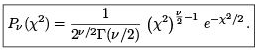(40)

where

ν = N - M.                 (41)

Finally, all the discussion of t parameters and their errors, is irrelevant if the curve does t the data. It is therefore essential to also calculate a "goodness of t parameter" Q. This is de ned to be the probability that one would get a value for X2 greater than or equal to the observed one by chance. In otherwords it is the area under the curve of Pν (X2 ) to the right of the observed value.
If X2 is less than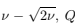will be close to 1 (so the t is very likely), whereas if X2 is much greater than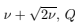will be close to zero (so the t is very unlikely).
An expression for Q can be found in terms of tabulated functions, since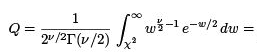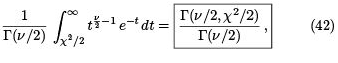Where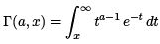(43)

is called the incomplete Gamma function. Note that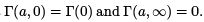159 docs

,

,

,

,

,

,

,

,

,

,

,

,

,

,

,

,

,

,

,

,

,

,

,

,

;